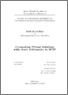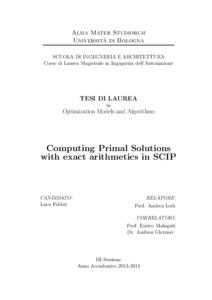# Computing primal solutions with exact arithmetics in SCIP.

Fabbri, Luca (2015) Computing primal solutions with exact arithmetics in SCIP. [Laurea magistrale], Università di Bologna, Corso di Studio in Ingegneria dell'automazione [LM-DM270]
Documenti full-text disponibili:Anteprima
Documento PDF

## Abstract

The research for exact solutions of mixed integer problems is an active topic in the scientific community. State-of-the-art MIP solvers exploit a floating- point numerical representation, therefore introducing small approximations. Although such MIP solvers yield reliable results for the majority of problems, there are cases in which a higher accuracy is required. Indeed, it is known that for some applications floating-point solvers provide falsely feasible solutions, i.e. solutions marked as feasible because of approximations that would not pass a check with exact arithmetic and cannot be practically implemented. The framework of the current dissertation is SCIP, a mixed integer programs solver mainly developed at Zuse Institute Berlin. In the same site we considered a new approach for exactly solving MIPs. Specifically, we developed a constraint handler to plug into SCIP, with the aim to analyze the accuracy of provided floating-point solutions and compute exact primal solutions starting from floating-point ones. We conducted a few computational experiments to test the exact primal constraint handler through the adoption of two main settings. Analysis mode allowed to collect statistics about current SCIP solutions' reliability. Our results confirm that floating-point solutions are accurate enough with respect to many instances. However, our analysis highlighted the presence of numerical errors of variable entity. By using the enforce mode, our constraint handler is able to suggest exact solutions starting from the integer part of a floating-point solution. With the latter setting, results show a general improvement of the quality of provided final solutions, without a significant loss of performances.

Abstract
Tipologia del documento
Tesi di laurea (Laurea magistrale)
Autore della tesi
Fabbri, Luca
Relatore della tesi
Scuola
Corso di studio
Indirizzo
Curriculum: Automation engineering
Ordinamento Cds
DM270
Parole chiave
SCIP, mixed integer programming, exact arithmetic, constraint handler, solution's accuracy, wireless network design problem
Data di discussione della Tesi
18 Marzo 2015
URI